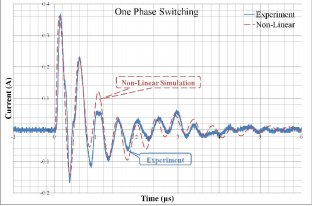Non-Linear Common-Mode Equivalent Circuit for Inverter-Fed Motor Drive Systems

Author(s):
Yizhanyi Tang, Satoshi Ogasawara, Masatsugu Takemoto (Hokkaido University)

This paper presents a discussion on common mode (CM) electromagnetic interference (EMI) in an inverter-fed motor drive system, and proposes a non-linear CM equivalent circuit. The effectiveness and necessity of
the non-linear element introduction are confirmed. The contributions of this paper can be summarized as follows:

• Possibility and rationality of non-linear resistance existing in the CM equivalent circuit model is confirmed. An equivalent circuit with non-linear element is proposed. Considering two aspects, only one phase switching and two phases switching, the necessity of non-linear equivalent circuit has been confirmed.
• In order to improve the accuracy of the equivalent circuit parameters and reduce errors, the optimization software modeFRONTIER is applied to identify the non-liner CM equivalent circuit. This conclusion can be proved that the introduction of non-linear resistance is efficiency and necessary.